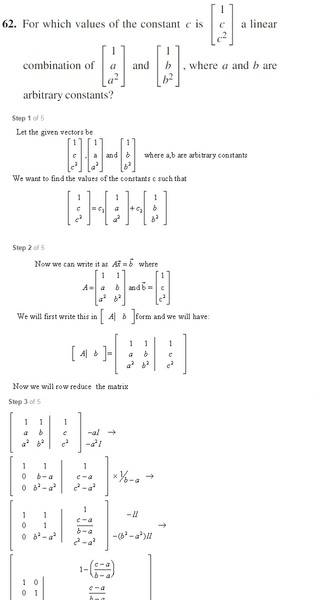# Linear Combination - Are these solutions wrong?I, II represent rows 1 and 2, respectively.

I am not agreeing with these solutions at step 3 of 5, where they multiply row 2 by 1/(b-a). Correct me if I'm wrong, but we do not know if b-a=0, so I do not think we can divide row II by (b-a) because of the potential of dividing by 0?

What do you guys think?

Mark44
MentorI, II represent rows 1 and 2, respectively.

I am not agreeing with these solutions at step 3 of 5, where they multiply row 2 by 1/(b-a). Correct me if I'm wrong, but we do not know if b-a=0, so I do not think we can divide row II by (b-a) because of the potential of dividing by 0?

What do you guys think?
I agree with you. From the given information, we can't say that b - a ≠ 0.